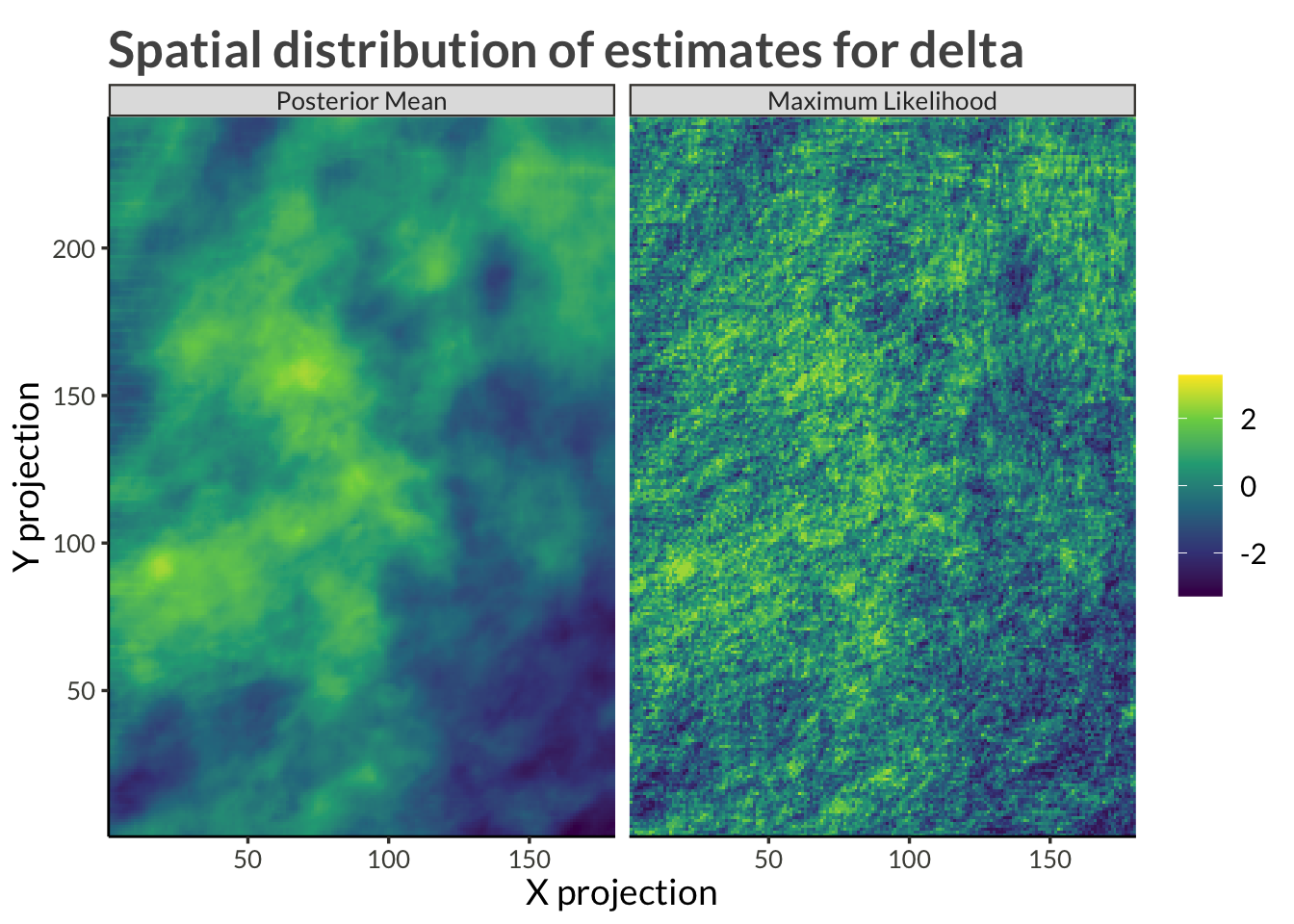# The Smooth Step

Code
library(bggjphd)
library(tidyverse)
library(bayesplot)
library(posterior)
library(GGally)
library(scales)
library(cowplot)
library(kableExtra)
library(arrow)
theme_set(theme_bggj())

The latent parameters, $$\psi$$, $$\tau$$, $$\phi$$, and $$\gamma$$, are given intrinsic random walk spatial priors, for example

\begin{aligned} \psi &\sim \mathcal N(\mathbf 0, \tau_\psi \cdot Q_u) \\ \sigma_\psi &= \frac{1}{\sqrt\tau_\psi} \\ \sigma_\psi &\sim \mathrm{Exp}(1) \end{aligned}

Here, $$Q_u$$ is defined by

$Q_u = R \otimes I + I \otimes R,$

where $$I$$ is the identity matrix and

$R = \begin{bmatrix} 1 & -1 & & & & & \\ -1 & 2 & -1 & & & & \\ & -1 & 2 & -1 & & & \\ & & \ddots & \ddots & \ddots & & \\ & & &-1 &2 &-1 & \\ & & & & -1 & 1\\ \end{bmatrix}.$

The results were obtained by running ms_smooth() in parrallel on four cores with four chains each run for 4000 samples. Half of those samples were designated as warm-up and so we have a total of 8000 samples from the posterior.

Code
theta_results <- read_parquet("data/theta_results.parquet") |>
as_draws_df()

n_iter <- theta_results |>
as_tibble() |>
pull(.iteration) |>
max()

# MCMC Diagnostics

## Trace plots

Code
theta_results |>
filter(.chain != 4) |>
filter(.iteration > 1) |>
mcmc_trace()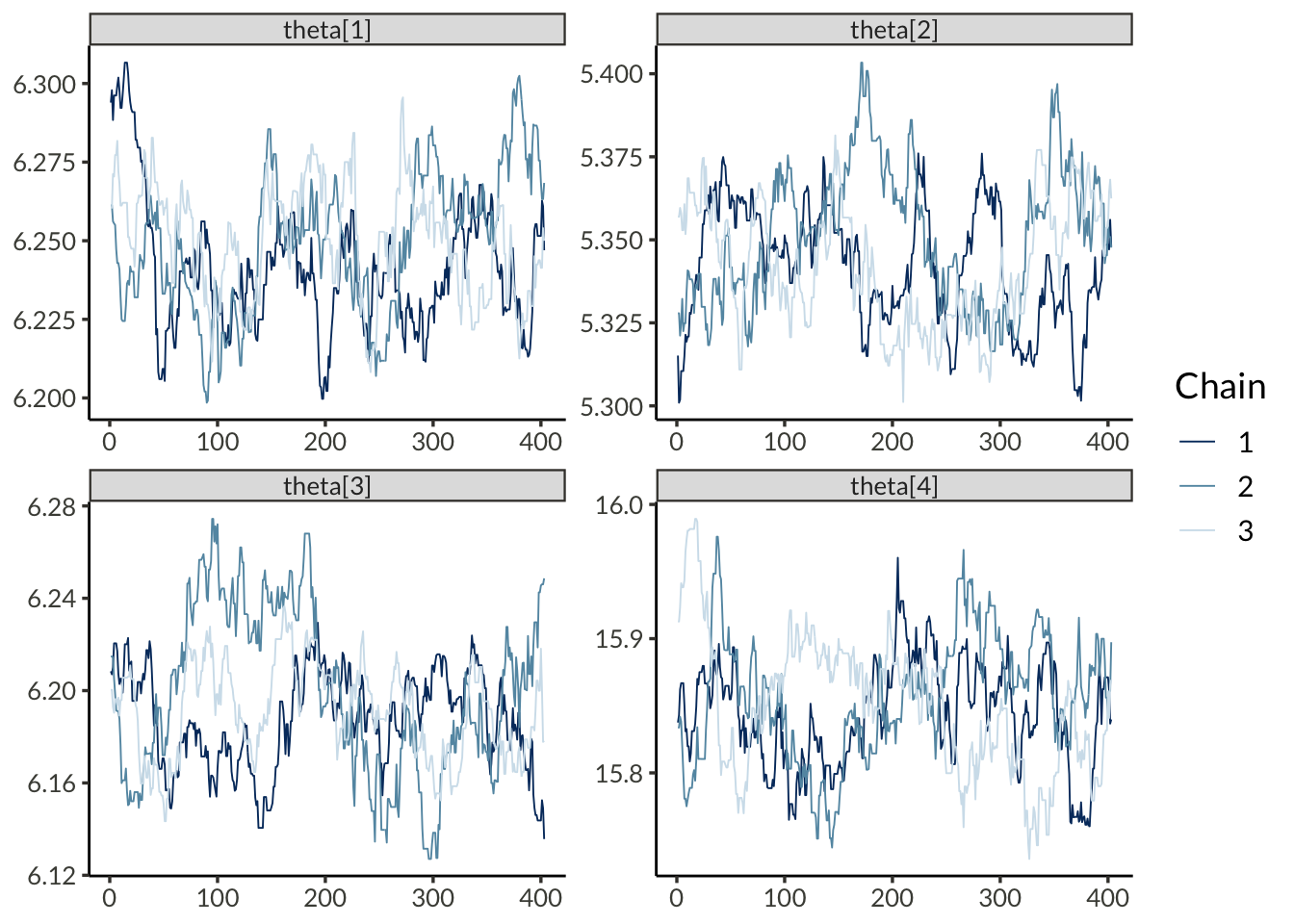## Autocorrelation functions

Code
theta_results |>
filter(.iteration > 1) |>
mcmc_acf_bar()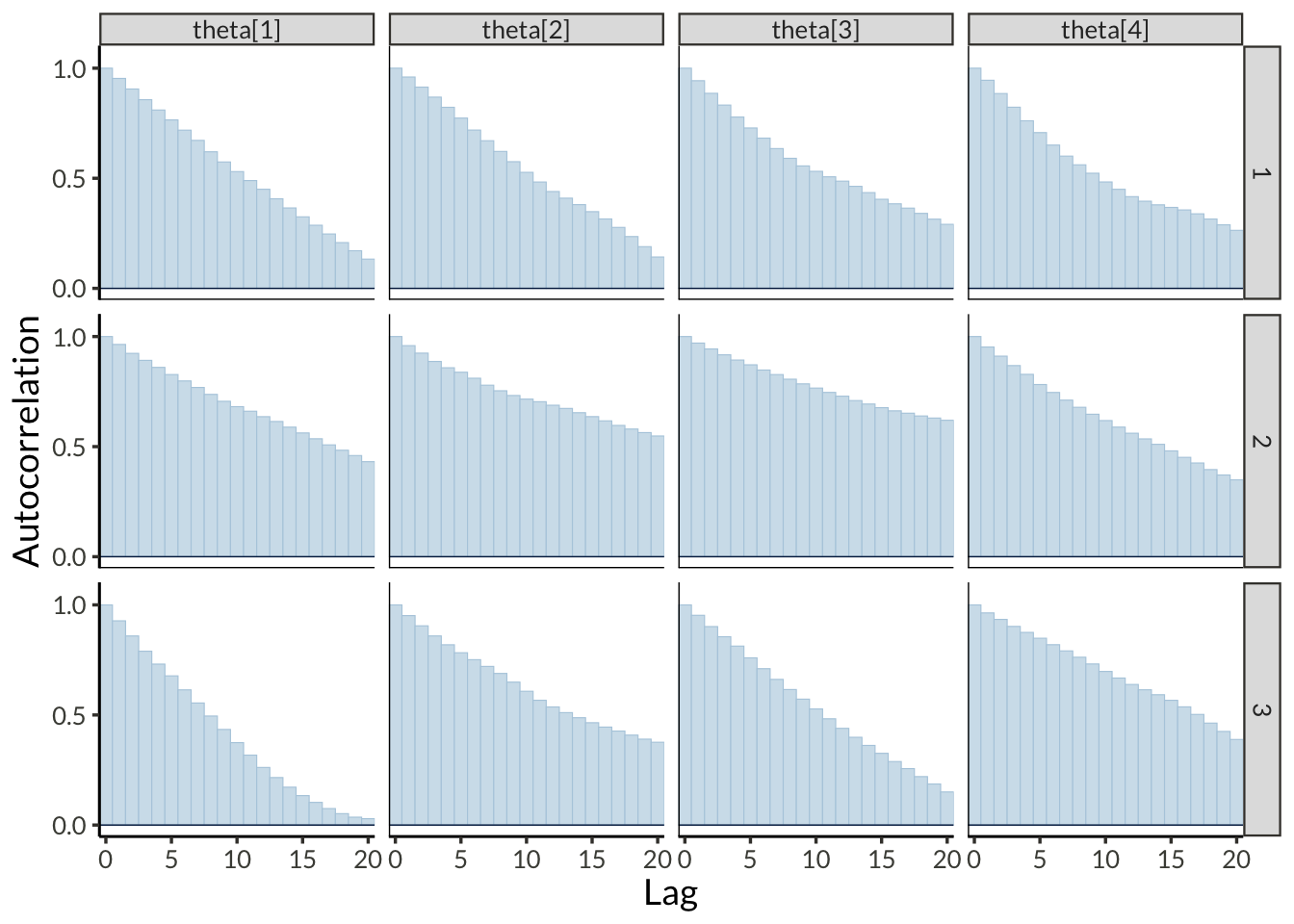## Acceptance probability

Code
theta_results |>
subset_draws("theta") |>
as_tibble() |>
rename(value = "theta") |>
group_by(.chain) |>
mutate(accept = 1 * (value != lag(value))) |>
ungroup() |>
ggplot(aes(.iteration, accept, group = .chain)) +
geom_smooth(method = "loess", span = 0.3, se = 0) +
scale_x_continuous(
expand = expansion()
) +
scale_y_continuous(
breaks = pretty_breaks(5),
labels = label_percent(),
expand = expansion()
) +
theme(
plot.margin = margin(t = 5, r = 35, b = 5, l = 5)
) +
coord_cartesian(ylim = c(0, 1)) +
labs(
x = "Iteration",
y = "Acceptance probability",
title = "Acceptance probability for theta"
)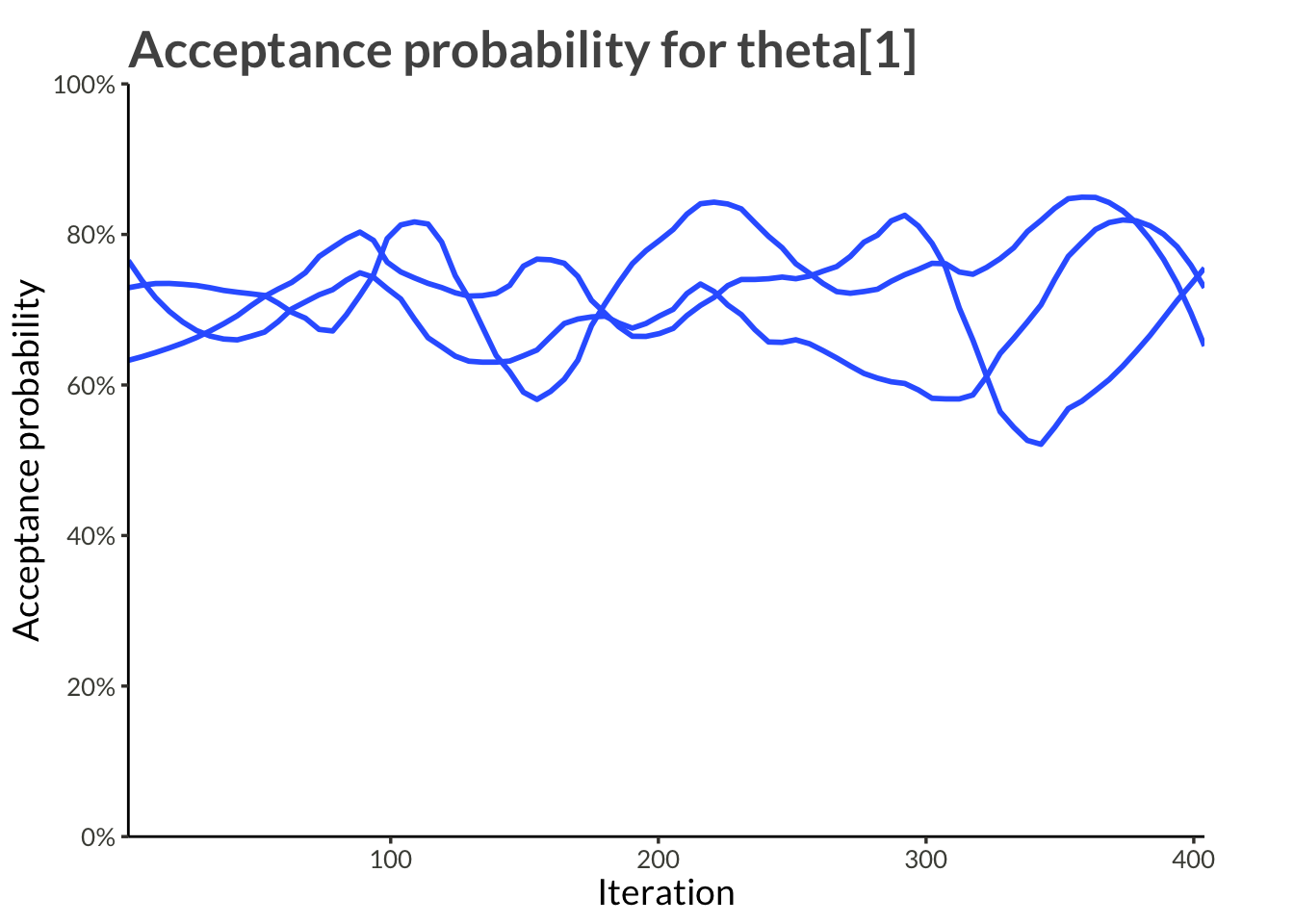# Parameters

## Hyperpriors

### Log precision scale

Code
theta_results |>
filter(.iteration > 1) |>
summarise_draws() |>
kable(digits = 3) |>
kable_styling(bootstrap_options = c("striped", "hover"))
variable mean median sd mad q5 q95 rhat ess_bulk ess_tail
theta 6.247 6.247 0.020 0.022 6.215 6.282 1.068 41.341 55.597
theta 5.345 5.345 0.019 0.022 5.315 5.375 1.056 30.657 53.149
theta 6.193 6.192 0.027 0.027 6.151 6.243 1.141 16.002 16.160
theta 15.850 15.851 0.045 0.047 15.775 15.923 1.172 14.069 65.501
Code
theta_results |>
filter(.iteration > 1) |>
mcmc_hist_by_chain(
)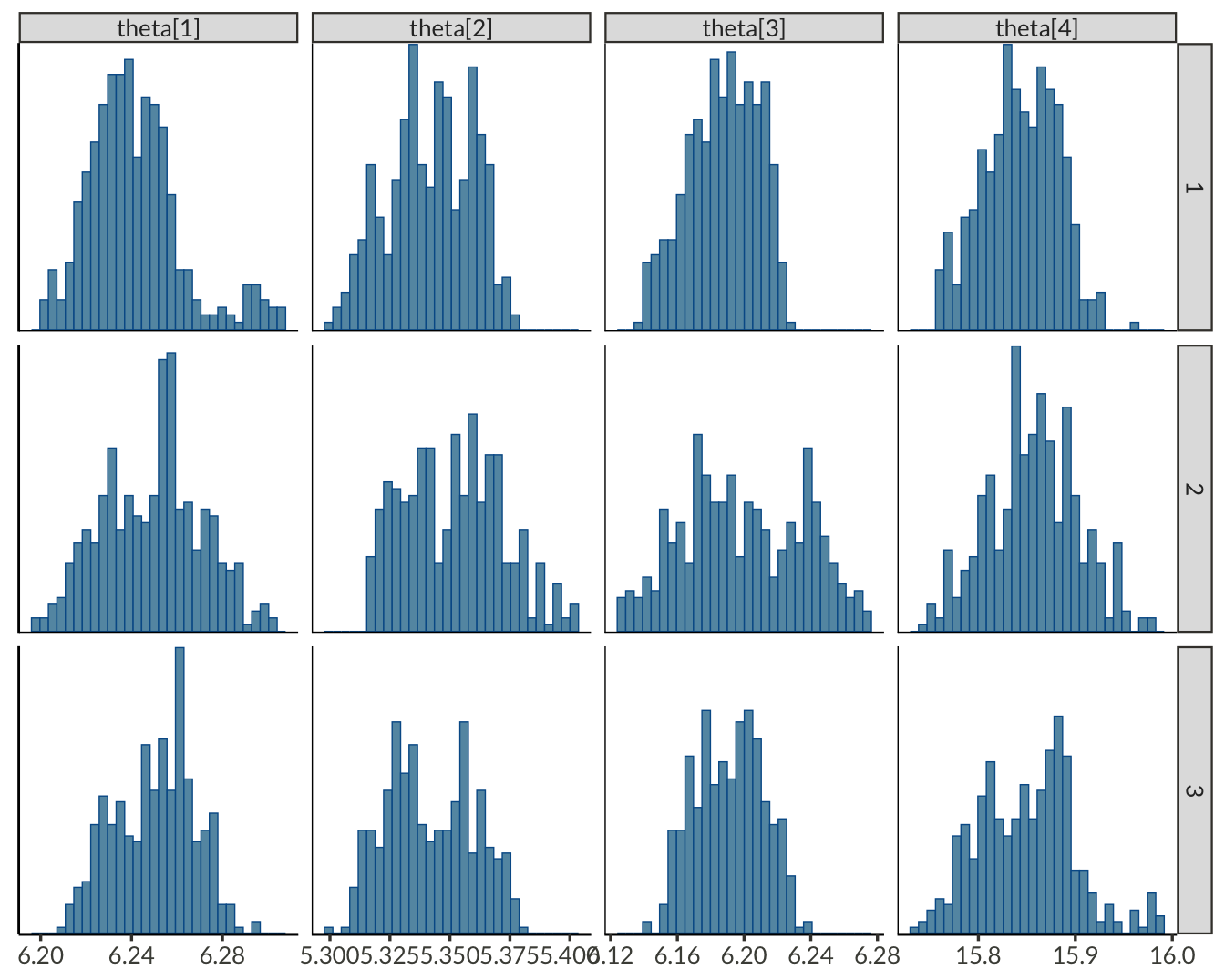### On standard deviation scale

Code
theta_results |>
filter(.iteration > 1) |>
summarise_draws() |>
kable(digits = 3) |>
kable_styling(bootstrap_options = c("striped", "hover"))
variable mean median sd mad q5 q95 rhat ess_bulk ess_tail
theta 6.247 6.247 0.020 0.022 6.215 6.282 1.068 41.341 55.597
theta 5.345 5.345 0.019 0.022 5.315 5.375 1.056 30.657 53.149
theta 6.193 6.192 0.027 0.027 6.151 6.243 1.141 16.002 16.160
theta 15.850 15.851 0.045 0.047 15.775 15.923 1.172 14.069 65.501
Code
theta_results |>
filter(.iteration > 1) |>
mcmc_hist_by_chain(
transformations = function(x) exp(-x/2)
)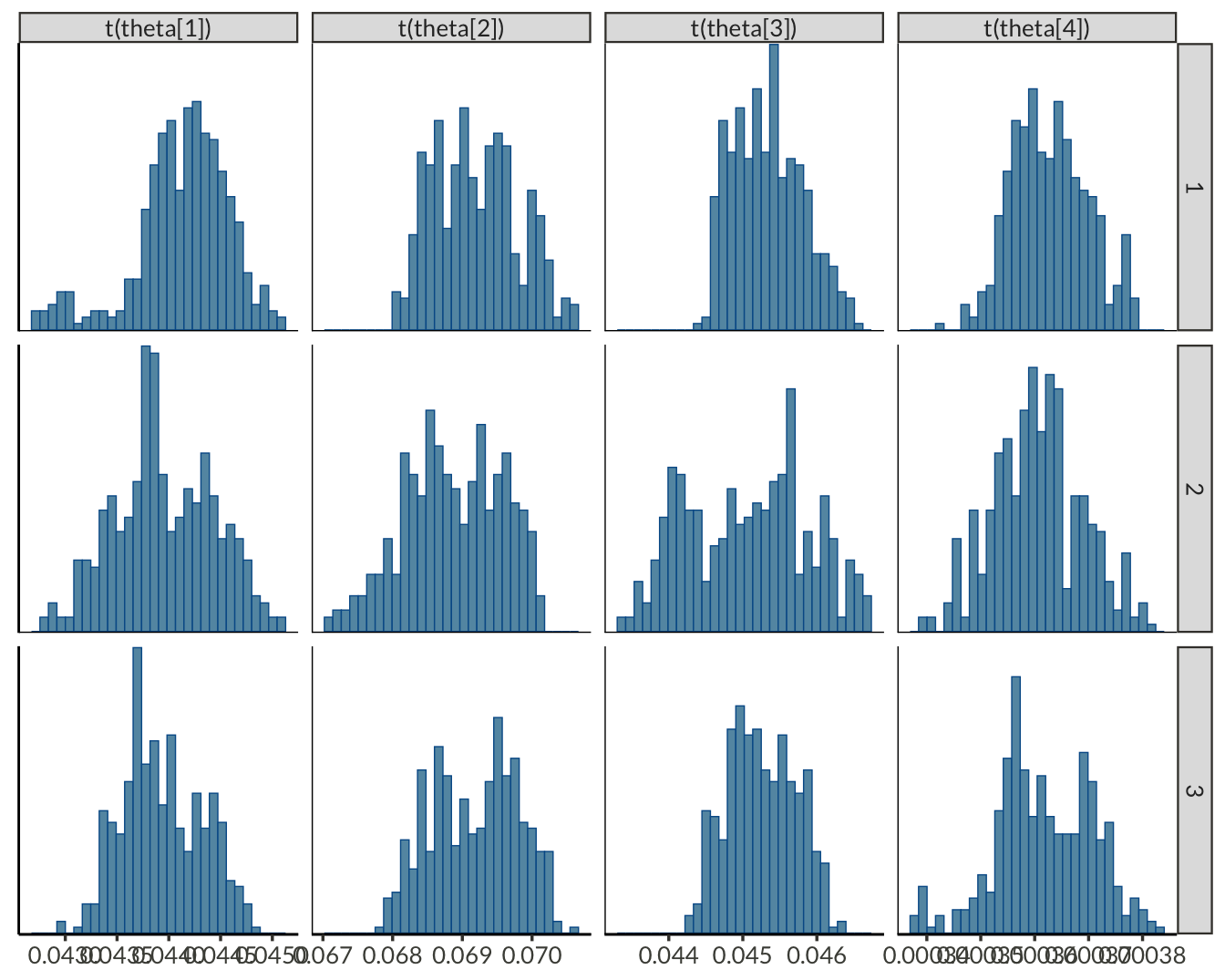## GEV Parameters

### Comparing ML and MCMC estimates

Code
station_results |>
pivot_longer(c(ml_estimate, mcmc_mean)) |>
mutate(
variable = fct_relevel(
factor(variable),
"psi", "tau", "phi", "gamma"
),
name = fct_recode(
factor(name),
"Maximum Likelihood" = "ml_estimate",
"Posterior Mean" = "mcmc_mean"
)
) |>
ggplot(aes(value)) +
geom_histogram() +
facet_wrap(vars(variable, name), ncol = 2, scales = "free_x") +
theme(
axis.line.y = element_blank(),
axis.text.y = element_blank(),
axis.ticks.y = element_blank()
) +
labs(
x = NULL,
y = NULL,
title = "Distributions of station parameters from ML and MCMC"
)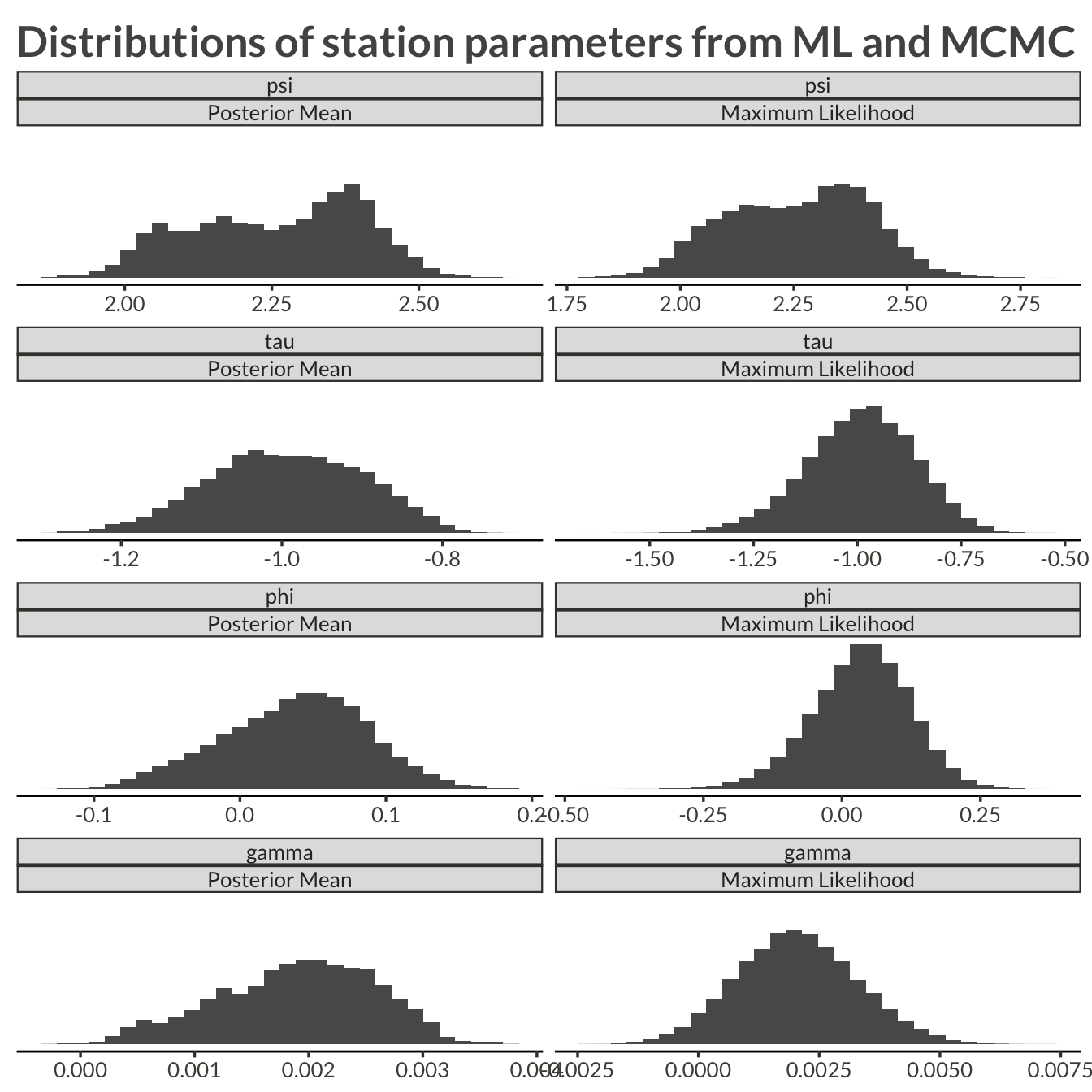Code
station_results |>
ggplot(aes(ml_estimate, mcmc_mean)) +
geom_abline(intercept = 0, slope = 1, lty = 2) +
geom_point(alpha = 0.1) +
facet_wrap("variable", scales = "free") +
labs(
x = "ML Estimate (Max step)",
y = "Posterior Mean (Smooth step)",
title = "Comparing estimates from the Max and the Smooth steps"
)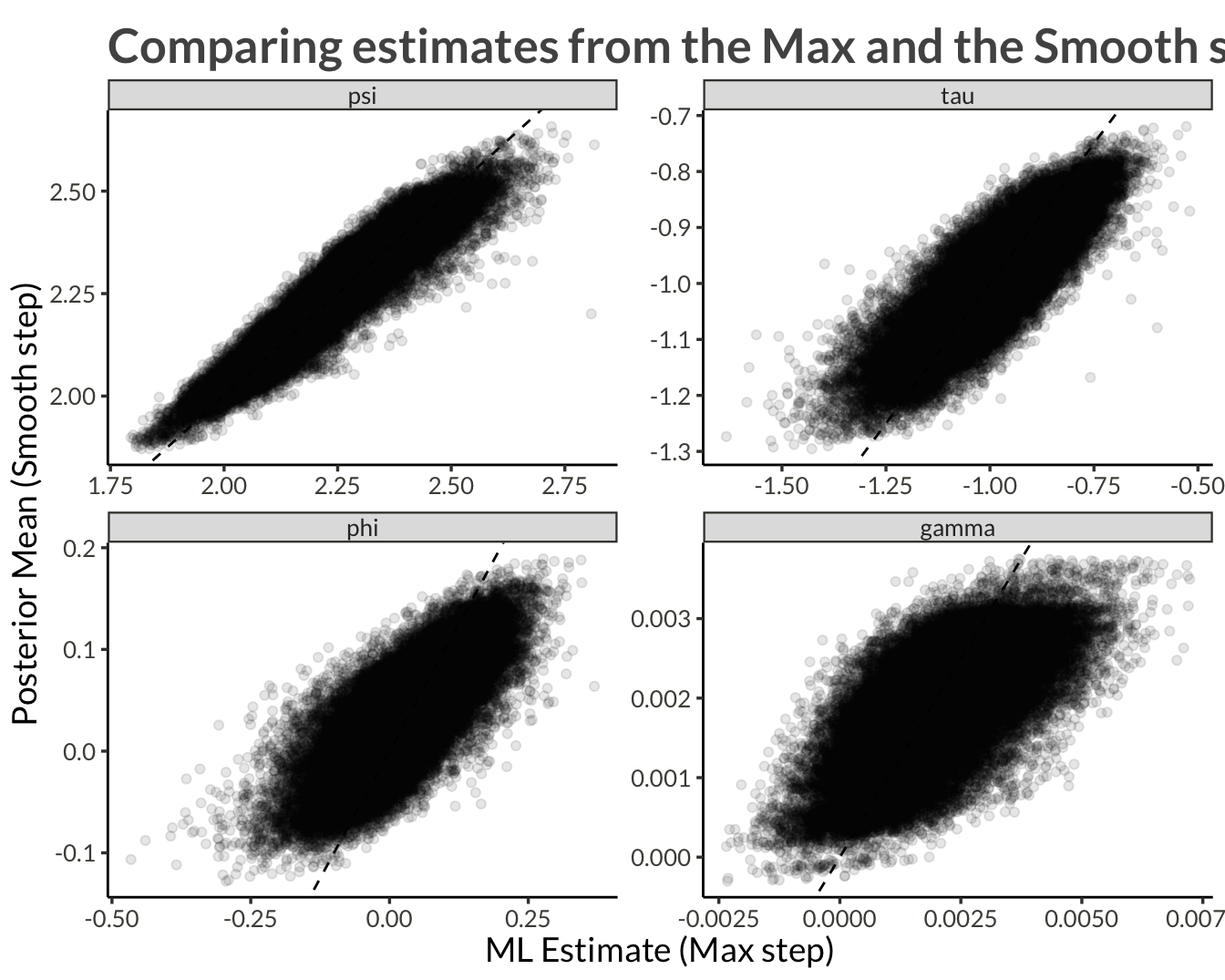Code
station_results |>
pivot_longer(c(ml_estimate, mcmc_mean)) |>
pivot_wider(names_from = variable) |>
mutate(
mu = exp(psi),
sigma = exp(psi + tau),
) |>
select(-(gamma:tau)) |>
pivot_longer(c(mu:delta), names_to = "variable") |>
pivot_wider() |>
ggplot(aes(ml_estimate, mcmc_mean)) +
geom_abline(intercept = 0, slope = 1, lty = 2) +
geom_point(alpha = 0.1) +
facet_wrap("variable", scales = "free") +
labs(
x = "ML Estimate (Max step)",
y = "Posterior Mean (Smooth step)",
title = "Comparing estimates from the Max and the Smooth steps"
)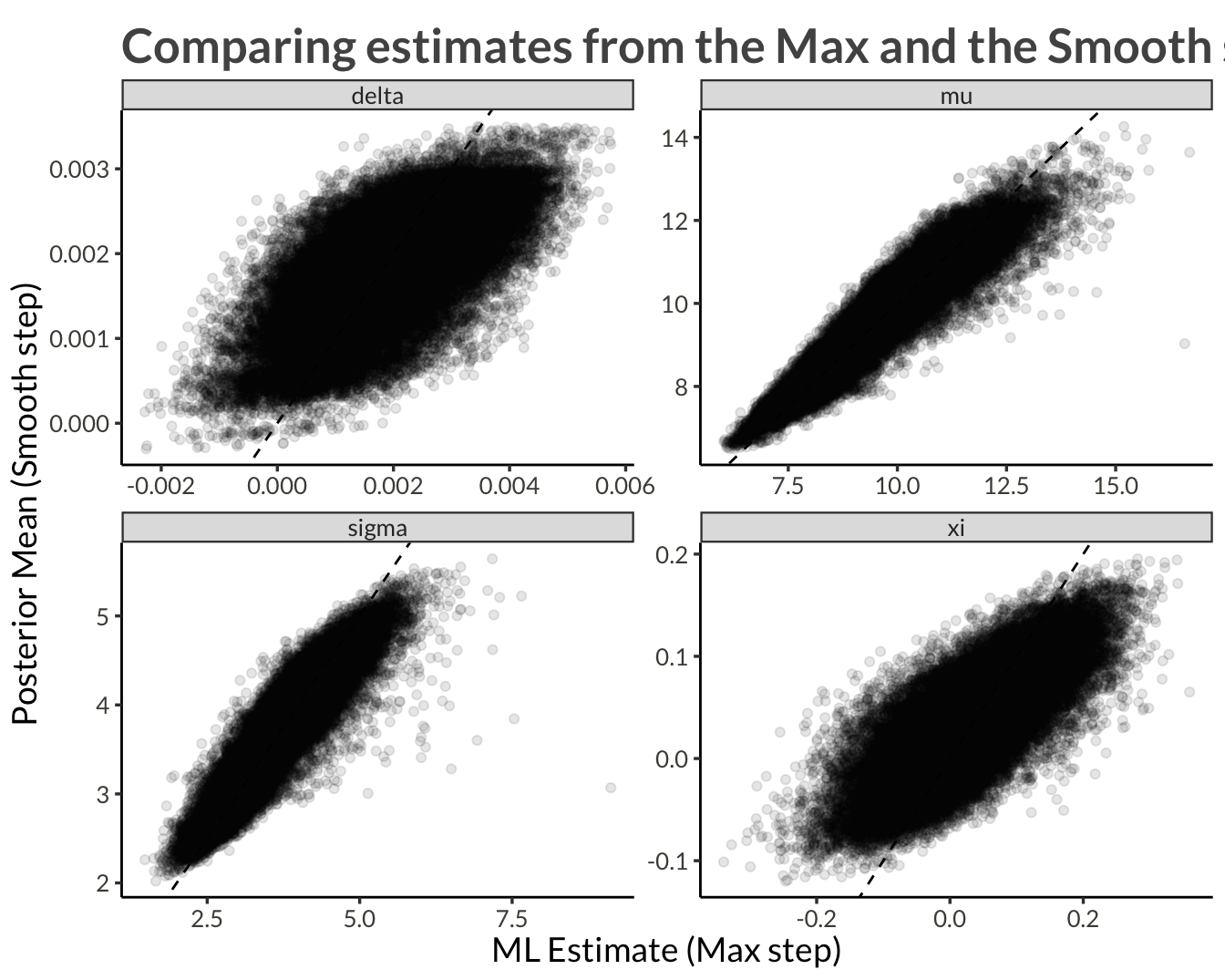### Spatial Distributions

Code
proj_plot <- function(data) {

title <- str_c(
"Spatial distribution of estimates for ", unique(data$variable) ) plot_dat <- data |> pivot_longer(c(ml_estimate, mcmc_mean)) |> mutate( name = fct_recode( factor(name), "Maximum Likelihood" = "ml_estimate", "Posterior Mean" = "mcmc_mean" ) ) |> group_by(name) |> mutate( value = (value - mean(value)) / sd(value) ) |> ungroup() |> mutate( value = case_when( name == "Posterior Mean" ~ value, value < quantile(value, 0.0025) ~ quantile(value, 0.0025), value > quantile(value, 0.9975) ~ quantile(value, 0.9975), TRUE ~ value ) ) min_val <- min(plot_dat$value)
max_val <- max(plot_dat\$value)

lim_range <- max(abs(min_val), abs(max_val))

limits <- c(-1, 1) * lim_range

plot_dat |>
ggplot(aes(proj_x, proj_y)) +
geom_raster(aes(fill = value)) +
scale_fill_viridis_c(limits = limits) +
facet_wrap("name", nrow = 1) +
coord_cartesian(expand = FALSE) +
labs(
title = title,
fill = NULL,
x = "X projection",
y = "Y projection"
)
}

#### Location

##### psi
Code
station_results |>
filter(variable == "psi") |>
proj_plot()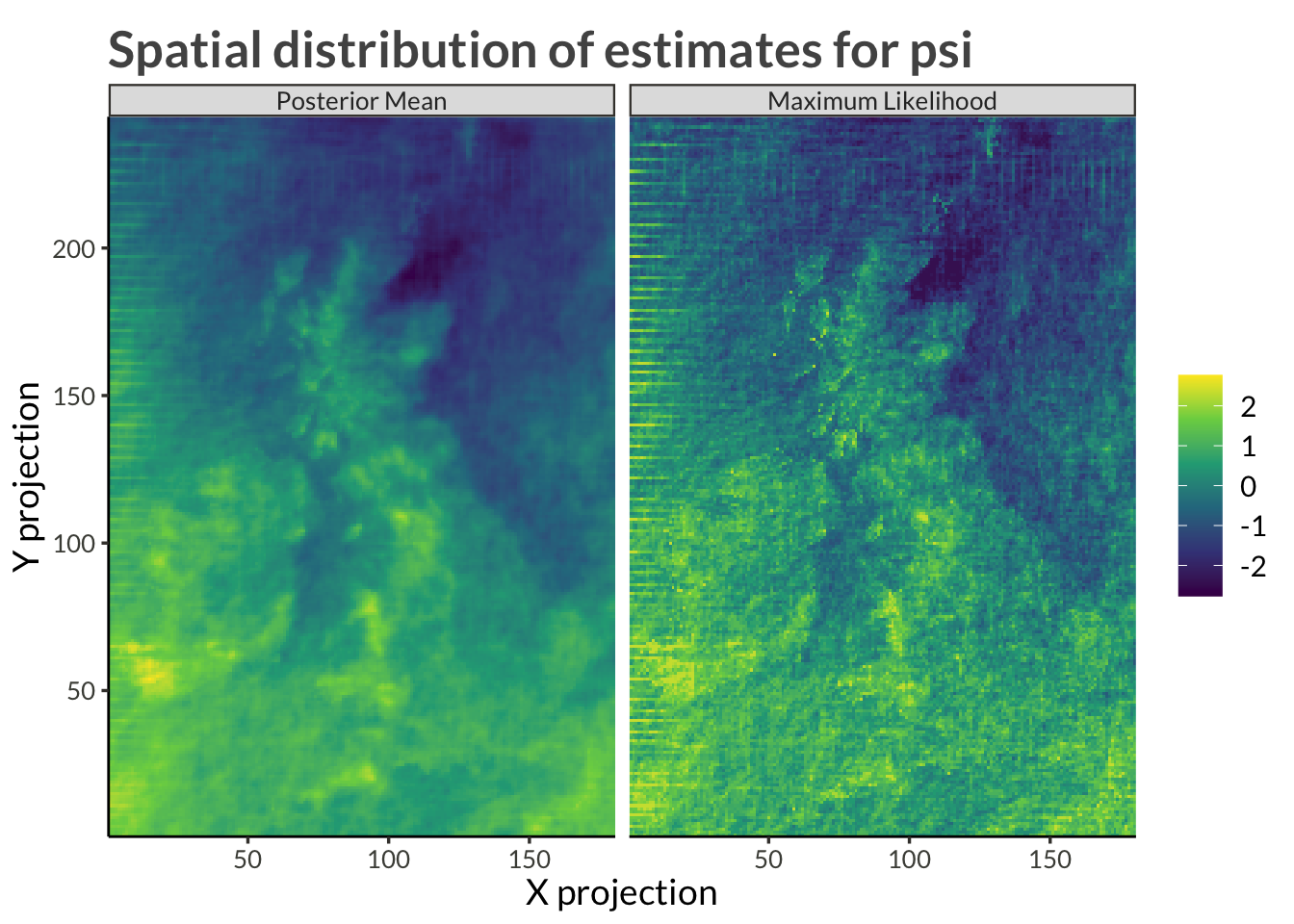##### mu
Code
station_results |>
filter(variable == "psi") |>
mutate(variable = "mu") |>
mutate_at(vars(ml_estimate, mcmc_mean), exp) |>
proj_plot()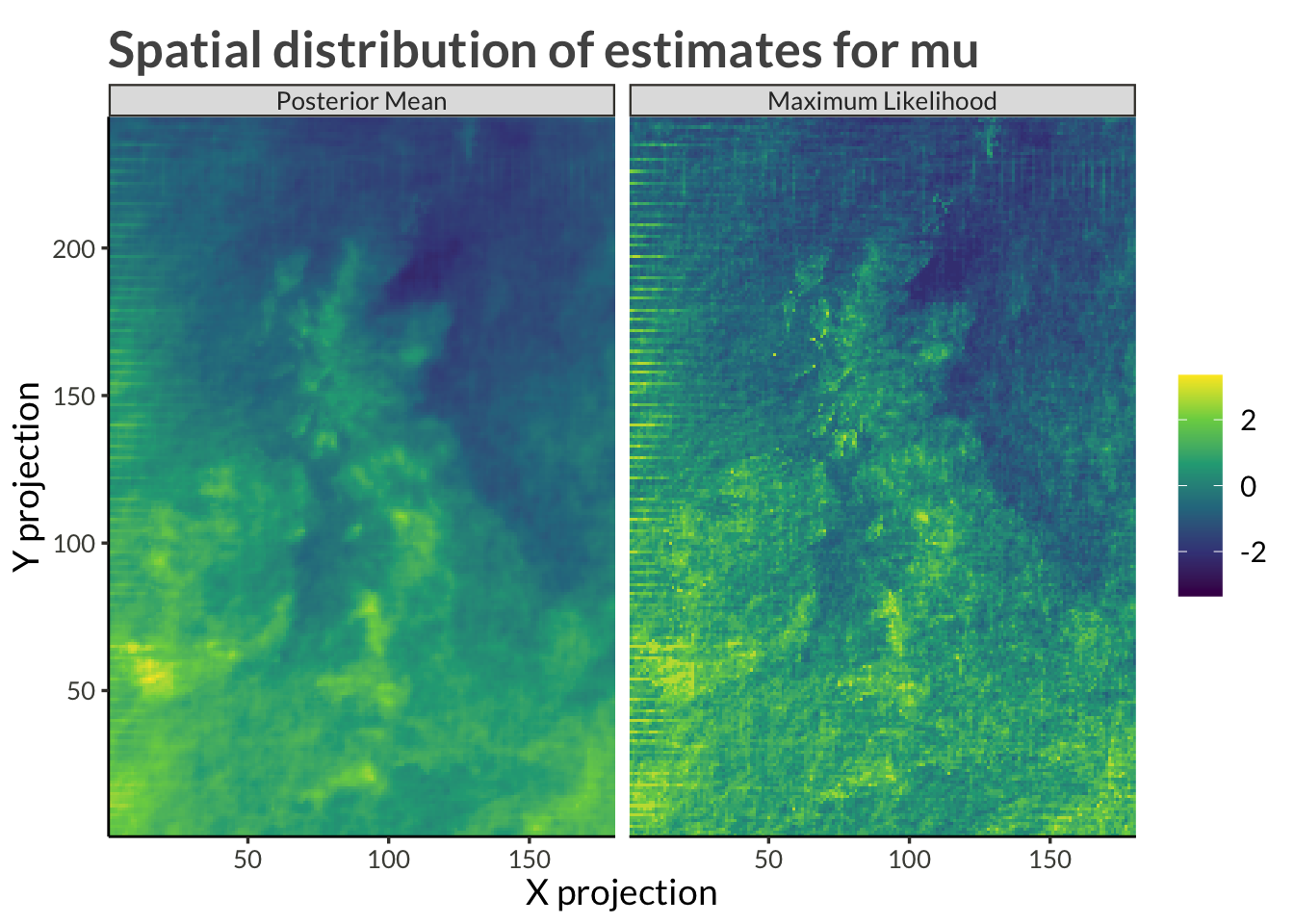#### Scale

##### tau
Code
station_results |>
filter(variable == "tau") |>
proj_plot()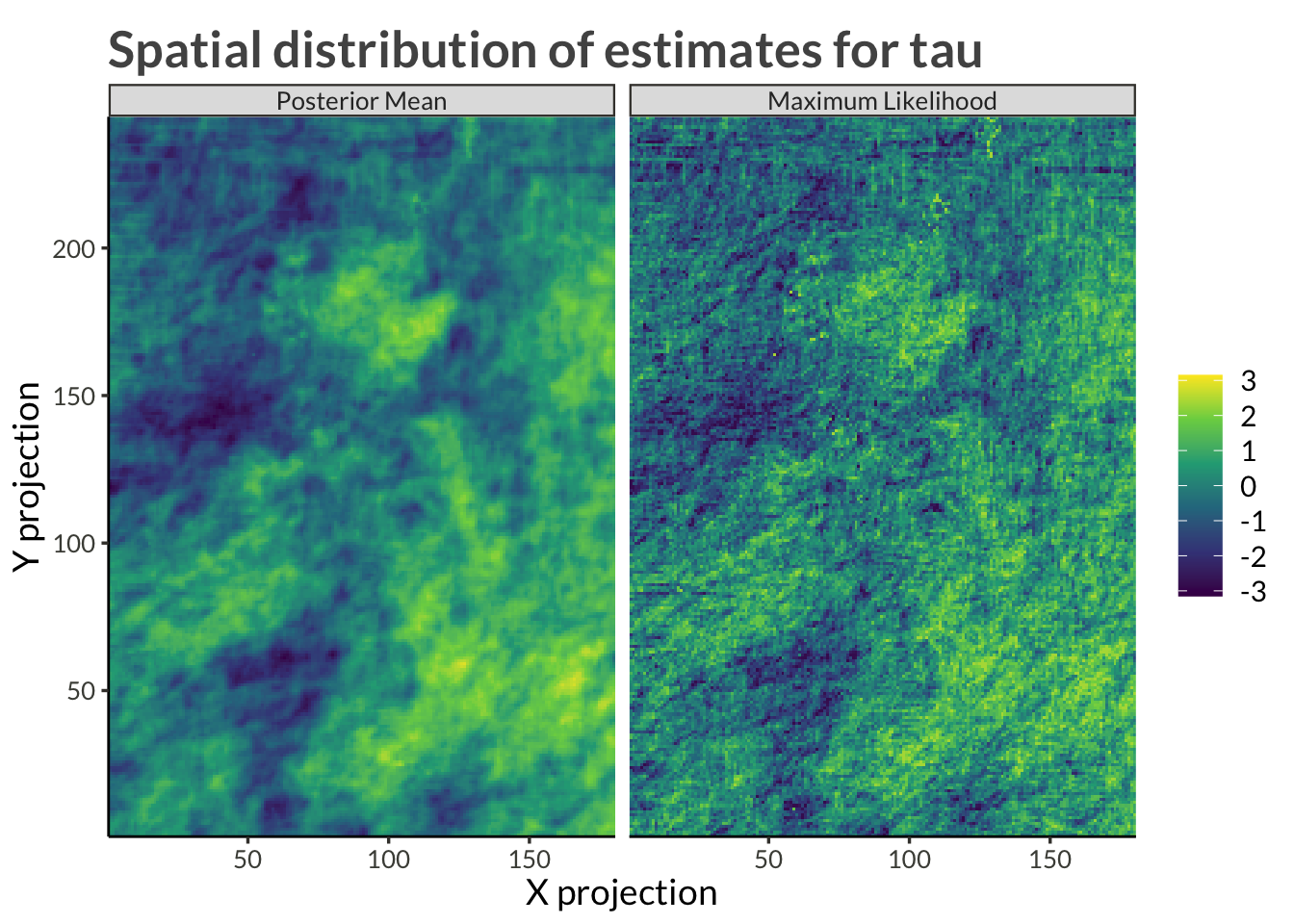##### sigma
Code
station_results |>
filter(variable %in% c("tau", "psi")) |>
pivot_longer(c(ml_estimate, mcmc_mean)) |>
pivot_wider(names_from = variable, values_from = value) |>
mutate(sigma = exp(tau + psi)) |>
select(-psi, -tau) |>
pivot_longer(c(sigma), names_to = "variable", values_to = "value") |>
pivot_wider() |>
proj_plot()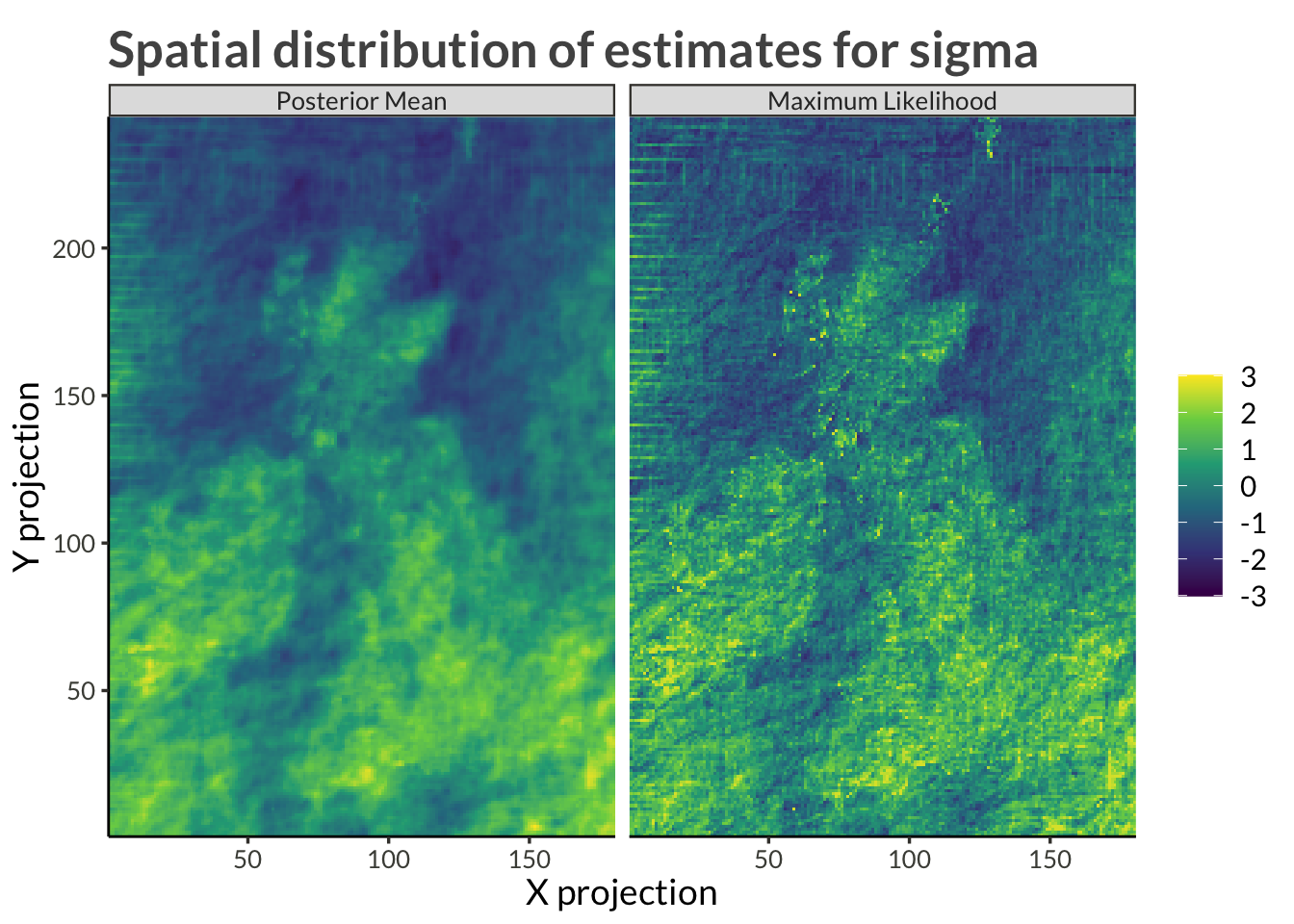#### Shape

##### phi
Code
station_results |>
filter(variable == "phi") |>
proj_plot()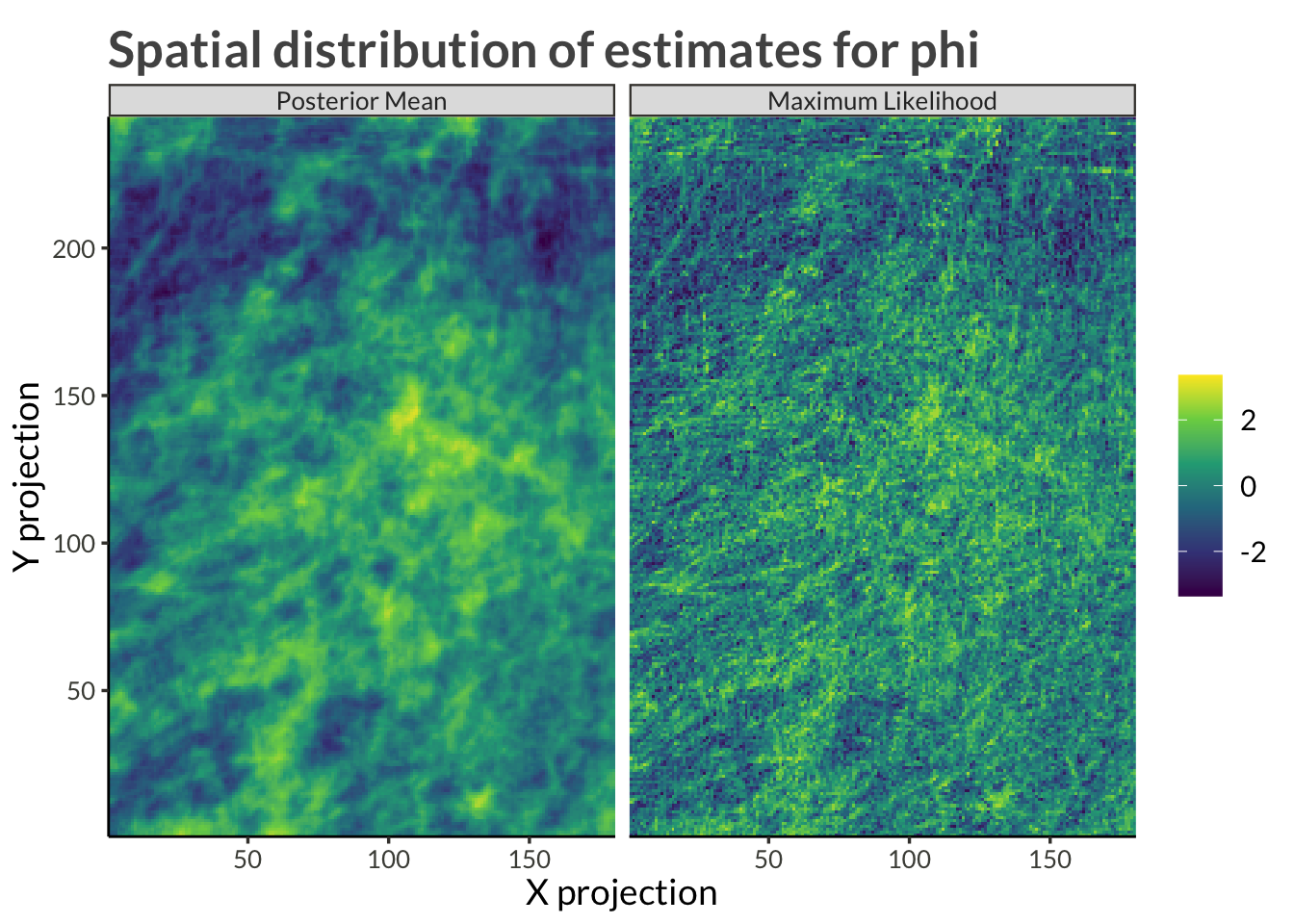##### xi
Code
station_results |>
filter(variable == "phi") |>
mutate(variable = "xi") |>
mutate_at(
vars(mcmc_mean, ml_estimate),
) |>
proj_plot()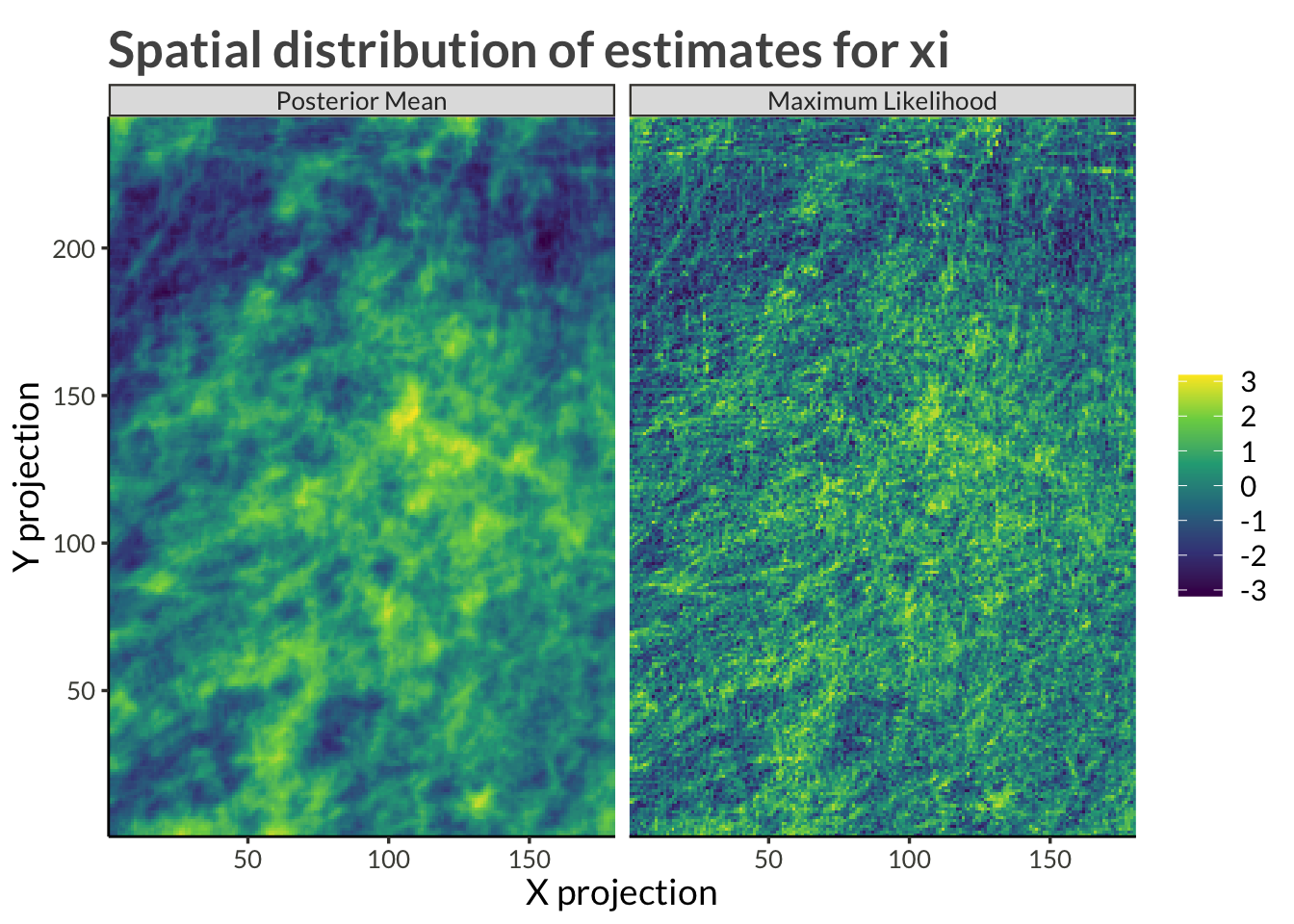#### Trend

##### gamma
Code
station_results |>
filter(variable == "gamma") |>
proj_plot()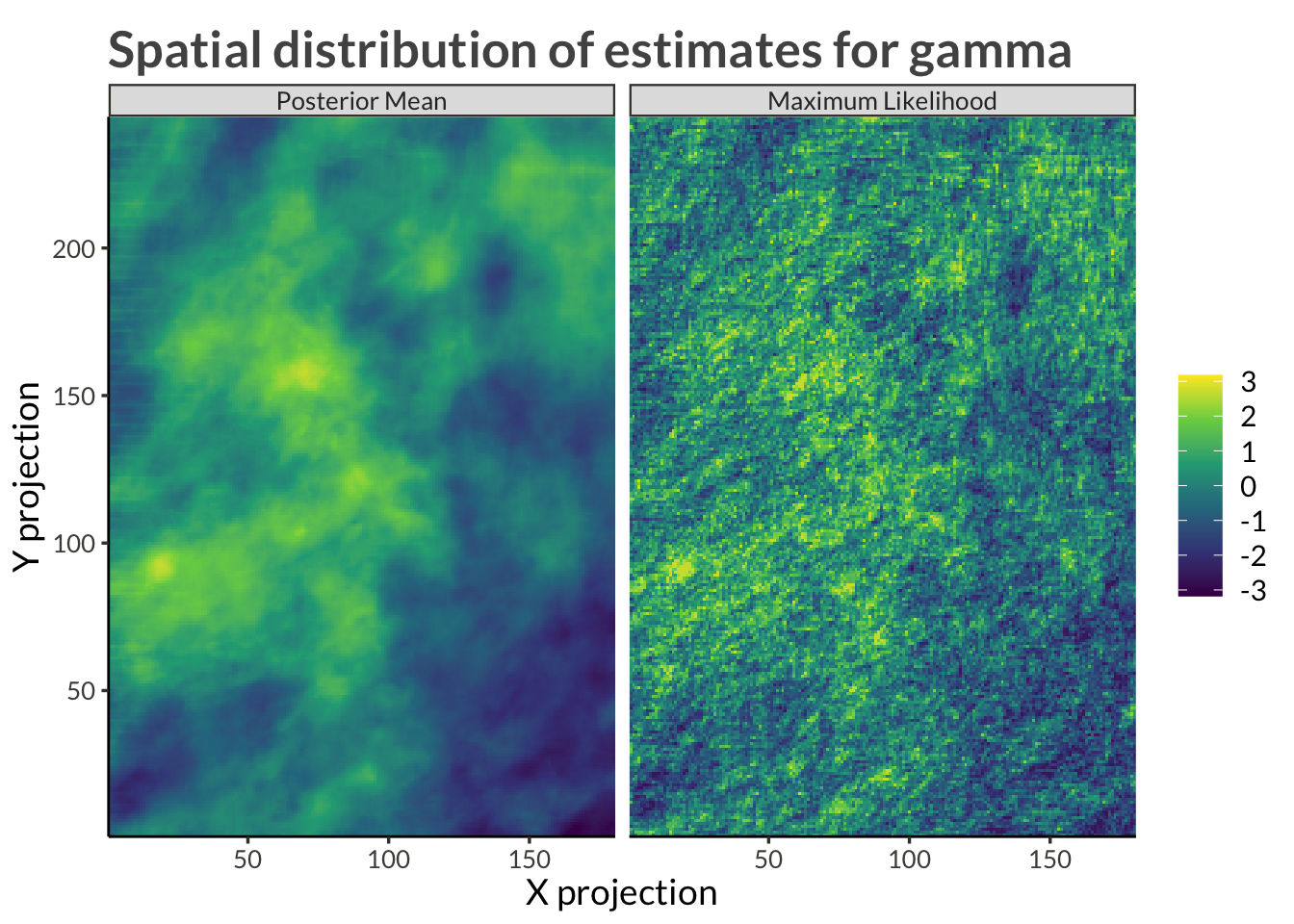##### Delta
Code
station_results |>
filter(variable == "gamma") |>
mutate(variable = "delta") |>
mutate_at(
vars(mcmc_mean, ml_estimate),
proj_plot()